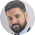# Ratio and Proportions : Tricks, questions and Formulae

Ratio and proportion is a very general topic for all bank exams. Mostly bank exams includes this topic in quantitative section.

Ratio is a quantity which represents the relationship between two similar quantities. It expresses a magnitude by which quantity is multiple of another one. Ratio is represented as 2:3 or 2/3. Here, numerator i.e. 2 is known as "ANTECEDENT" and denominator i.e. 3 is known as "CONSEQUENT".

If antecedent is more than the consequent, then it is known as improper ratio and if less ,then it is known as proper ratio.

### Some important points:

1) If ratio is written as A:B, it is said to be a linear form and in case it is written as A/B, it is said to be fractional form.

2) Ratio does not have any unit. It is mere number.

3) The equality of two ratios is known as proportion i.e. a/b = c/d

If a/b = c/d , then it is also equal to a+c/b+d

Invertendo : If a/b = c/d , then b/a = d/c

Alterendo : If a/b = c/d , then a/c = b/d

Componendo : If a/b = c/d , then a+b/b = c+d/d

Dividendo : If a/b = c/d , then a-b/b = c-d/d

Componendo and Dividendo : If a/b = c/d , then a+b/a-b = c+d/c-d

4) If a/b = b/c = c/d =...... so on, then a,b,c,d... are in G.P.

Proof: Let a/b = b/c = c/d =k
c= dk, b= ck, a= bk
Therefore, b= dk^2 and a= dk^2
All are in G.P.

5) If a>b and same positive number is added to each term, then ratio decreases.
For example: a/b = 4/3 = 1.3, If 2 is added to each term, then a/b = 4+2/3+2 = 6/5 = 1.2
Therefore, ratio decreases.

6) If a<b and same positive number is added to each term, then ratio increases.
For example: a/b = 3/4 = 0.7, If 2 is added to each term, then a/b = 3+2/4+2 = 5/6 = 0.8
Therefore, ratio increases.

7) If we multiply or divide any number, there will be no effect on ratio.

8) Let a:b is a ratio

a^2:b^2 is duplicate ratio of a:b
a^3:b^3 is triplicate ratio of a:b
a^1/2:b^1/2 is sub-duplicate ratio of a:b
a^1/3:b^1/3 is sub-triplicate ratio of a:b

9) Proportions i.e. a:b = c:d
a and d are known to be extremes
b and c are known to be means.

10) In a:b :: c:d, d is fourth proportional to a,b and c.

11) If x is third proportional to a,b then it is written as a:b :: b:x.

Problem: 94 is divided into two parts in such a way that the fifth part of second are in ratio 3:4. The first part is?
Solution: Let these parts are A and B

A/5 = 3 , This implies, A = 15
B/8 4 B 32
1st part = 94*15 = Rs30
47#### What's trending in BankExamsToday

Smart Prep Kit for Banking Exams by Ramandeep Singh - Download here1.i was very happy to see shortcuts for aptitude in this site..bt i'm unable to see things clearly...only some words are displayed here and there..i dont know y this is happening...very disappointed to see that...if the problem is with ur site clear it immedietly...plz...

1.Problem fixed, check now

2.check the last problem. i am unable to understand it and also i am unable to solve it

3.unable to understand the problem.....plz help..

Thanks for commenting. Follow us on Telegram. Search BankExamsToday